# The only ideas that can be used include: area ABCI-RA2(A+B+C-lpi), the Pythagorean theorem: Cos c cos a cos b. Vectors-dot product cross product, sin A-sin a/sin c; cos A-cos a sin b/sin c; spher...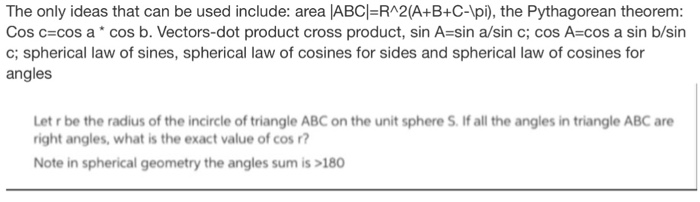The only ideas that can be used include: area ABCI-RA2(A+B+C-lpi), the Pythagorean theorem: Cos c cos a cos b. Vectors-dot product cross product, sin A-sin a/sin c; cos A-cos a sin b/sin c; spherical law of sines, spherical law of cosines for sides and spherical law of cosines for angles Let r be the radius of the incircle of triangle ABC on the unit sphere S. If all the angles in triangle ABC are right angles, what is the exact value of cos? Note in spherical geometry the angles sum is >180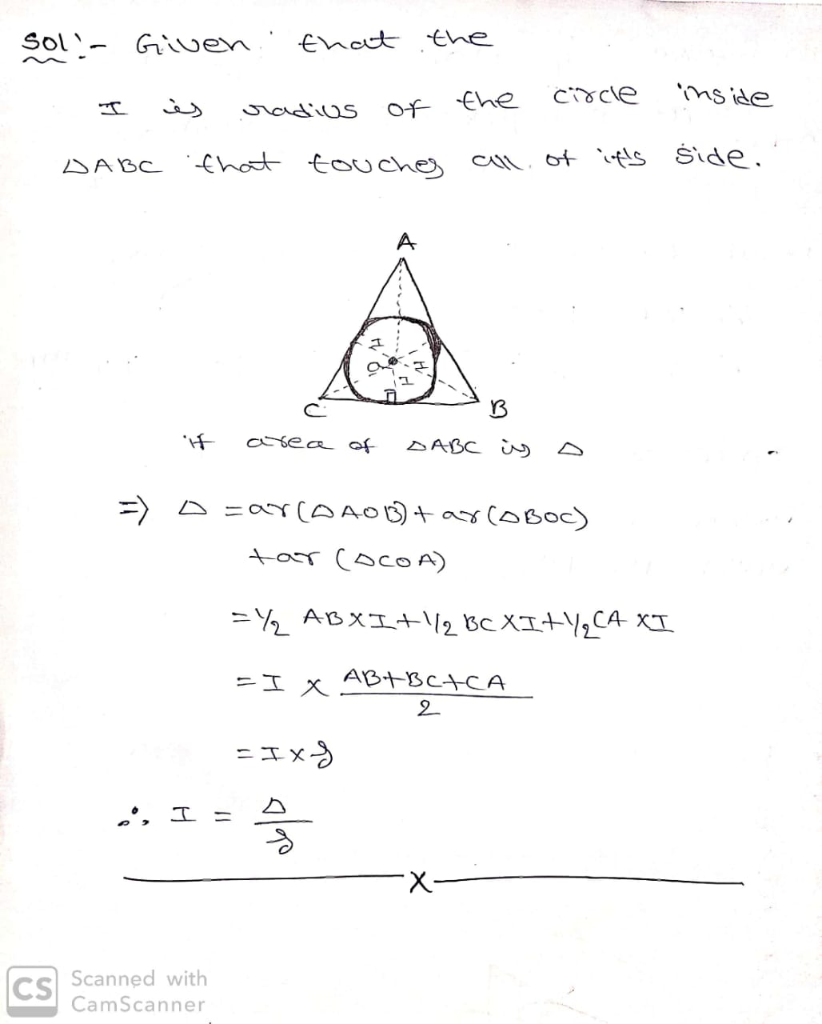##### Add Answer of: The only ideas that can be used include: area ABCI-RA2(A+B+C-lpi), the Pythagorean theorem: Cos c cos a cos b. Vectors-dot product cross product, sin A-sin a/sin c; cos A-cos a sin b/sin c; spher...
Similar Homework Help Questions
• ### 1. Let ABCDE be a regular pentagon on the unit sphere S with each side equal to s and each angle equal to 4pi/5. Find the exact value of cos a. Noticed that as in Euclidean geometry a regular pe...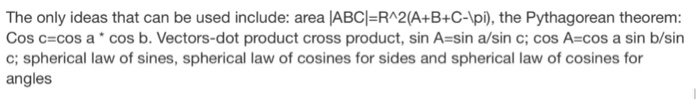1. Let ABCDE be a regular pentagon on the unit sphere S with each side equal to s and each angle equal to 4pi/5. Find the exact value of cos a. Noticed that as in Euclidean geometry a regular pentagon called a spear can be inscribed in a spherical circle The only ideas that can be used include: area ABC-RA2(A+B+C-Ipi), the Pythagorean theorem: Cos c-cos a cos b. Vectors-dot product cross product, sin A-sin a/sin c; coS A-COs a sin...

• ### Do not use I=delta/S!!! Use law of cosines Here is the question: Let r be the radius of the incircle of triangle ABC on the unit sphere S. If all the angles in triangle ABC are right angles, wha...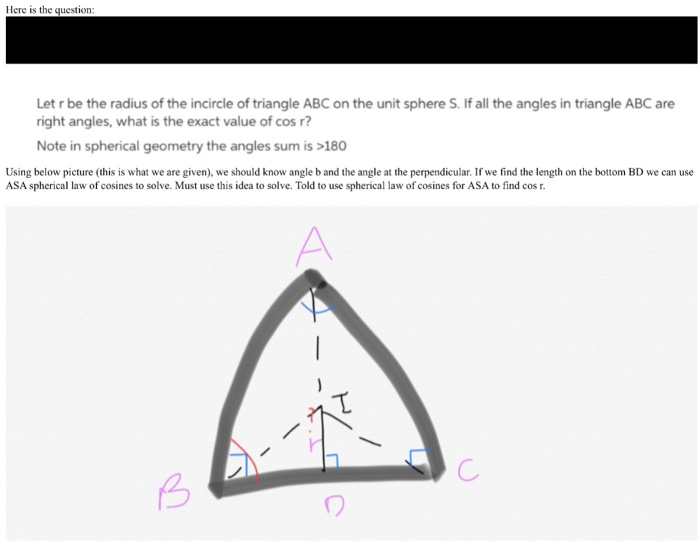Do not use I=delta/S!!! Use law of cosines Here is the question: Let r be the radius of the incircle of triangle ABC on the unit sphere S. If all the angles in triangle ABC are right angles, what is the exact value of cos r? Note in spherical geometry the angles sum is>180 Using below picture (this is what we are given), we should know angle b and the angle at the perpendicular. If we find the length on...

• ### My p1i = 6408.41640768       p2i = 4439.33266 Here is your task. Make a ...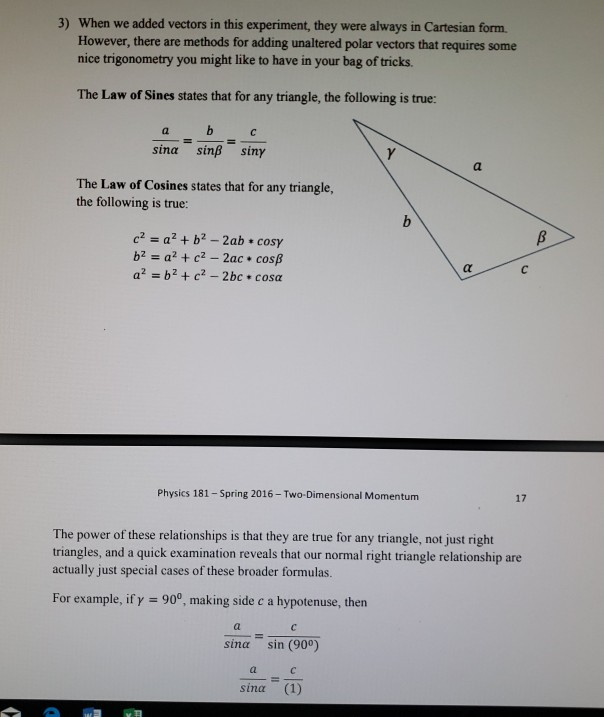my p1i = 6408.41640768       p2i = 4439.33266 Here is your task. Make a diagram of the initial momentum vectors adding to the total momentum vector, �" = �W," + �:,". (This will look like the right side of your Vector Plotting diagram you did in lab.) Use the Law of Sines and the Law of Cosines to add them to find the magnitude and angle of �". Do this without switching to Cartesian vectors or making any right triangles. (You...

• ### Let the angles of a triangle be ?, ?, and ?, with opposite sides of length a, b, and c,...

Let the angles of a triangle be α, β, and γ, with opposite sides of length a, b, and c, respectively. Use the Law of Sines to find the remaining sides. (Round youranswers to one decimal place.)α = 70°; γ = 46°; b = 15a=?c=?Let the angles of a triangle be α, β, and γ, with opposite sides of length a, b, and c, respectively. Use the Law of Cosines and the Law of Sines to find theremaining parts of...

• ### An) a) Find the magnitude of both vectors. b) Find dot product and cross product of both vectors ...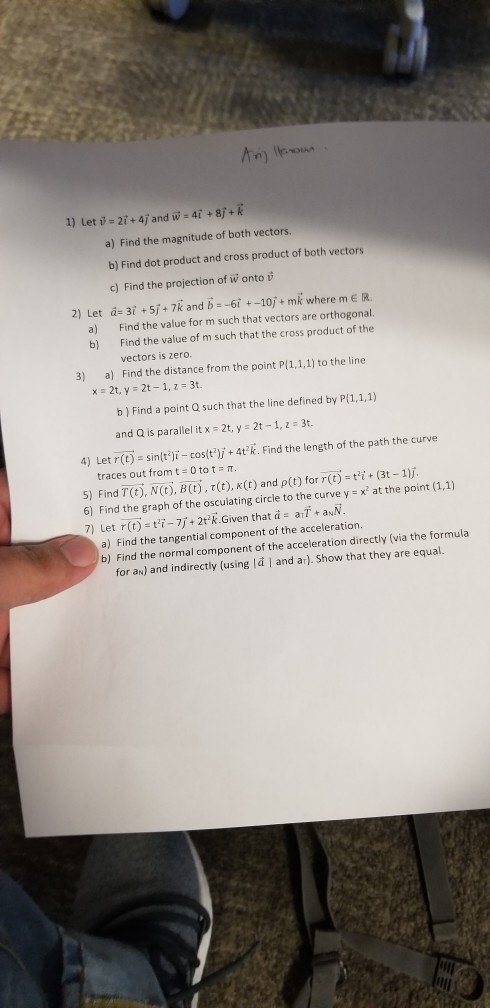pls answer 4,5,6 and 7 An) a) Find the magnitude of both vectors. b) Find dot product and cross product of both vectors c) Find the projection of w onto v 2) Let а:31 + 5, + 7k and b--6r +-10, + mk where m e R. a) Find the value for m such that vectors are orthogonal b) Find the value of m such that the cross product of the vectors is zero 3) a) Find the distance from...

• ### 2. Let A = (cos, sin and B = (cos, sin) be two vectors on the x-y plane. Let C = (cos, sin be another non-zero ve...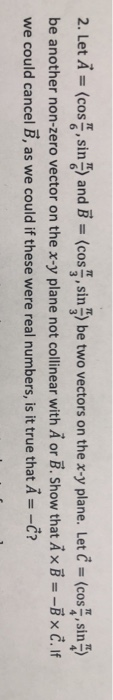2. Let A = (cos, sin and B = (cos, sin) be two vectors on the x-y plane. Let C = (cos, sin be another non-zero vector on the x-y plane not collinear with A or B. Show that Ax B = -Bx C. If we could cancel B, as we could if these were real numbers, is it true that A= -Č? 2. Let A = (cos, sin and B = (cos, sin) be two vectors on the x-y...

• ### Let the angles of a triangle be , ?, and ?, with opposite sides of length a, b, and c,...

Use the Law of Sines to find the remaining sides. (Round your answers to one decimal place.)a = 70°; ß = 30°; a = 33b=?c=?Use the Law of Cosines to find the remaining side and one of the other angles. (Round your answers to one decimal place.)? = 110°; a = 12; b = 16c=?a=?Use the Law of Cosines to find the remaining side and one of the other angles. (Round your answers to one decimal place.)ß = 32°; a...

• ### I am studying for my GRE exam into grad school and it's been a very long time since I've done geometry

I am studying for my GRE exam into grad school and it's been a very long time since I've done geometry. I have a problem to which I need to solve for the area of a triangle but I do not have the base. I do have all angles but I cannot remember how to convert angles into their corresponding line segments. Any help on this matter would be greatly appreciated. Thank you. Kaytee If you have all the angles,...

• ### Let the angles of a triangle be , ?, and ?, with opposite sides of length a, b, and c,...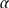Let the angles of a triangle be , β, and γ, with opposite sides of length a, b, and c, respectively. Use the Law of Cosines and the Law of Sines to find theremaining parts of the triangle. (Round your answers to one decimal place.)a = 5; b = 5; c = 9a =?β =?γ=?Let the angles of a triangle be , β,and γ, with opposite sides of length a, b, and c, respectively. Use the Law of Sines to...

• ### In triangle ABC if <A and <B are acute angles and sin 10/13 , what is the value of cos A

In triangle ABC if <A and <B are acute angles and sin 10/13 , what is the value of cos A?

Need Online Homework Help?